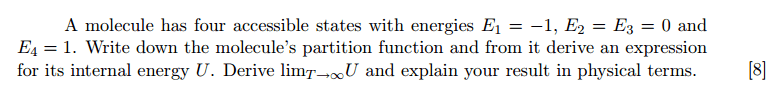# Partition function of simple system

## Homework StatementA molecule has 4 states of energy -1, 0,0 and 1. Find its partition function and limit of energy as T → ∞.

## The Attempt at a Solution

$$Z = \sum_r e^{-\beta E} = e^{-\beta} + 2 + e^{\beta}$$

$$U = -\frac{\partial ln(Z)}{\partial \beta} = \frac{e^{-\beta} - e^{\beta}}{e^{-\beta} + 2 + e^{\beta}}$$

As ##T→\infty##, ##exp(-\beta) \approx 1 - \beta## and ##exp(\beta) \approx 1 + \beta##.

Thus,
$$U \approx \frac{(1-\beta) - (1+\beta)}{2 + (1+\beta) + (1-\beta)} = -\frac{\beta}{2} = -\frac{1}{2kT}$$

The equipartition theorem should take over with Energy = 4 * (1/2)kT = 2kT = 2/β.
But instead i'm getting -β/2.

## Answers and Replies

bumpp

Ammm, okay...

Your calculation is right, at least I got the same result for ##U##.

my comment about the equipartition theorem: My experiences are that you really have to master thermodynamics to completely understand this theorem. Lots of results can be "guessed" if you truly understand the concept. I was never that good at it therefore I always had to do the long calculations.
Ok, now to tell something that is actually useful:

from http://chemwiki.ucdavis.edu/Physical_Chemistry/Statistical_Mechanics/Equipartition_Theorem (Degrees of freedom):
"The law of equipartition of energy states that each quadratic term in the classical expression for the energy contributes ½kBT to the average energy."

Let's take a molecule of ideal gas for example: One molecule has in fact ##6## degrees of freedom. ##3## of them precisely describe it's position and are called coordinates (x,y,z), the other ##3## are of course components of momentum (note that momentum is quadratic in energy ##E_k=\frac{p^2}{2m}##). Each component of momentum therefore contributes ##\frac{1}{2}kT##, so the average energy of molecule of ideal gas is ##\frac{3}{2}kT##.

I guess all I am trying to say is that you have no quadratic degrees of freedom and therefore your calculation using equipartition theorem is wrong.

ps: Keep in mind that I never mastered that theorem. I hope I didn't just make a fool out of myself.

try expanding the exponential in different form

Exp(-x) = 1/ exp(x) = 1/ (1+x)

try in this form and show what you get, i hope this work